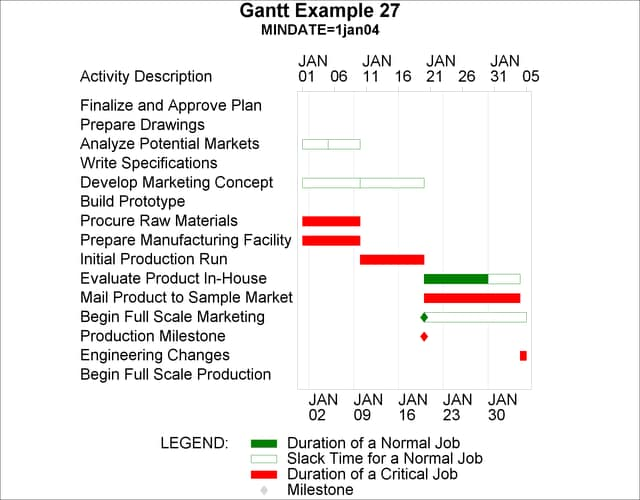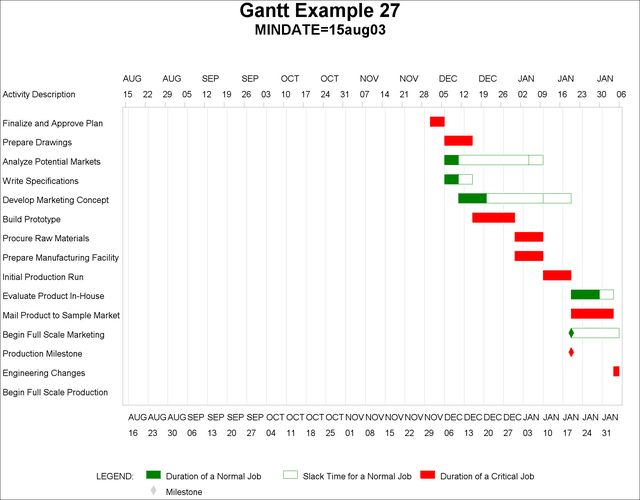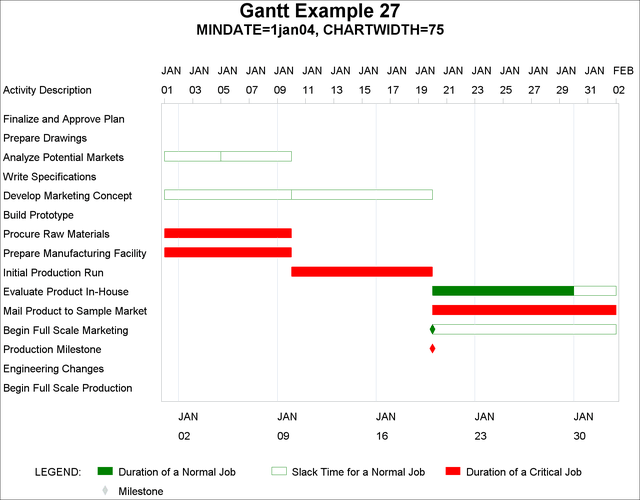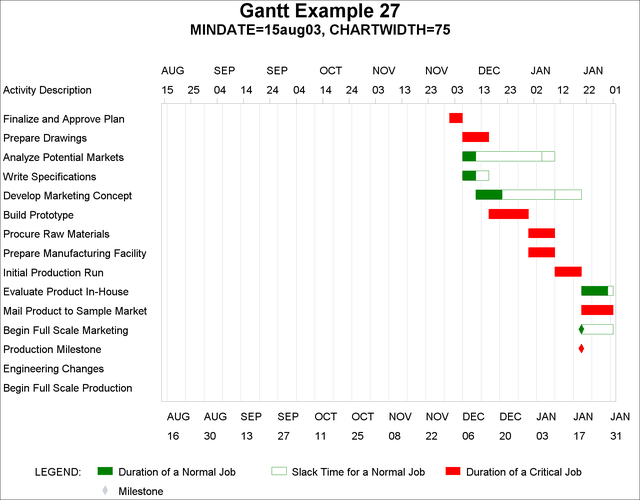### Example 8.27 Using the CHARTWIDTH= Option

This example illustrates the use of the CHARTWIDTH= option to create Gantt charts that are consistent in appearance. The data set used in this example is the `SAVE` data set created in Example 8.6.

Gantt charts are first produced using different values of the MINDATE= option, and without specifying the CHARTWIDTH= option. Output 8.27.1 shows a Gantt chart using MINDATE='1jan04', and Output 8.27.2 shows a Gantt chart using MINDATE='15aug03'. Notice that the chart in Output 8.27.2 has a much larger chart area than the chart in Output 8.27.1, and the 'Activity Description' column is compressed and rather difficult to read.

```title h=2 'Gantt Example 27';
title2 h=1.5 'MINDATE=1jan04';
proc gantt data=save;
chart / mindate='1jan04'd maxdate='1feb04'd
dur=days nojobnum compress height=2.0
ref='2jan04'd to '2feb04'd by week
reflabel;
id descrpt;
run;
```
```title h=2 'Gantt Example 27';
title2 h=1.5 'MINDATE=15aug03';
proc gantt data=save;
chart / mindate='15aug03'd maxdate='1feb04'd
dur=days nojobnum compress height=2.0
ref='16aug03'd to '2feb04'd by week
reflabel;
id descrpt;
run;
```

Output 8.27.1: Without the CHARTWIDTH= Option (MINDATE=1Jan04)Output 8.27.2: Without the CHARTWIDTH= Option (MINDATE=15Aug03)The same charts are now plotted with the CHARTWIDTH= option. The specification CHARTWIDTH=75 indicates that the chart is rescaled so the axis area is 75% of the chart width and the text area is 25% of the chart width. Therefore, specifying CHARTWIDTH=75 for both charts gives the two charts a consistent appearance. The output is shown in Output 8.27.3 and Output 8.27.4.

```title h=2 'Gantt Example 27';
title2 h=1.5 'MINDATE=1jan04, CHARTWIDTH=75';
proc gantt data=save;
chart / mindate='1jan04'd maxdate='1feb04'd
dur=days nojobnum compress height=2.0
ref='2jan04'd to '2feb04'd by week
reflabel chartwidth=75;
id descrpt;
run;
```
```title h=2 'Gantt Example 27';
title2 h=1.5 'MINDATE=15aug03, CHARTWIDTH=75';
proc gantt data=save;
chart / mindate='15aug03'd maxdate='1feb04'd
dur=days nojobnum compress height=2.0
ref='16aug03'd to '2feb04'd by week
reflabel chartwidth=75;
id descrpt;
run;
```

Output 8.27.3: Using the CHARTWIDTH= Option (MINDATE=1Jan04)Output 8.27.4: Using the CHARTWIDTH= Option (MINDATE=15Aug03)